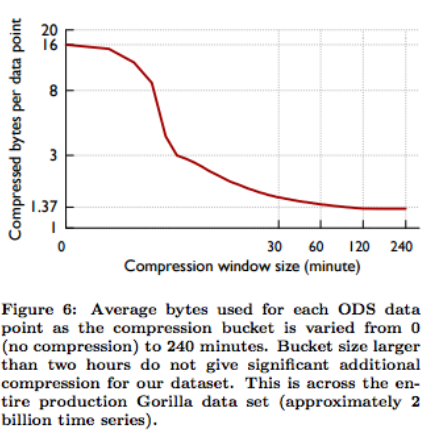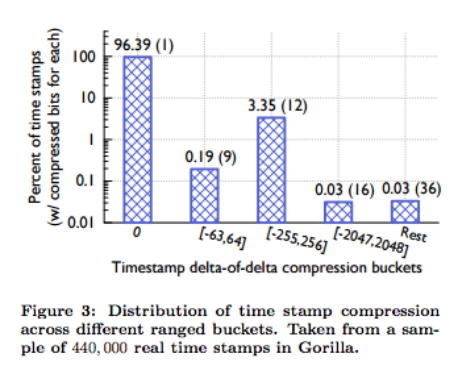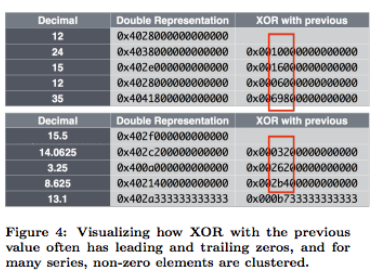(come from Paper)

## 導讀:

### 什麼是 Gorilla ?

Gorilla 是 Facebook 開發的時間序列資料庫．其實市場上已經有很多的時間序列資料庫 (HBase on TSDB(time-series database)) ，為什麼還需要自己開發一個呢?

• 資料的儲存過於龐大
• 查詢的延遲過長

• 針對 timestamp 的壓縮
• 分析並且針對資料的壓縮
• 放在記憶體 (in-memory databse)

### 那麼 Gorilla 比起一般的時間序列資料庫究竟有多強大呢?

• 73 倍的 Query Latency 減少
• 14 倍的 Query Throughput 增加(come from Paper)

### 12倍的壓縮比？ 那麼大的壓縮比是如何做到?

• 連續性資料
• 在比較小的取樣時間中，每筆資料間的變化不大

### 壓縮的演算法: 關於時間資料的壓縮

• $t_0$ => 02: 00 : 00
• $t_1$ => 02: 01 : 02
• $t_2$ => 02: 02 : 02
• $t_3$ => 02: 03 : 02

• $t_0$ => 02: 00 : 00 (跟之前差距 0)
• $t_1$ => 02: 01 : 02 (跟之前差距 62 秒)
• $t_2$ => 02: 02 : 02 (跟之前差距 60 秒)
• $t_3$ => 02: 03 : 02 (跟之前差距 60 秒)

• $t_0$ => 02: 00 : 00 (跟之前差距 0)
• $t_1$ => 02: 01 : 02 (跟之前差距 62 秒)
• $t_2$ => 02: 02 : 02 (跟之前差距 60 秒，跟上一個差距的差異 -2 秒 )
• $t_3$ => 02: 03 : 02 (跟之前差距 60 秒,跟上一個差距的差異 0)(Pic: from Morning Paper )

• $t_0$ => 02: 00 : 00 => D = 0, tag bit 0.
• $t_1$ => 02: 01 : 02 => D = 62, tag bit 10, value 62 (011 1110) 總共 9 bits
• $t_2$ => 02: 02 : 02 => D = -2, tag bit 10, value -2 (111 1101) 總共 9 bits
• $t_3$ => 02: 03 : 02 => D = 0, tag bit 0 總共 1 bit(come from Paper)

### 壓縮的演算法: 關於時間資料的壓縮 (程式碼)

func (s *Series) Push(t uint32, v float64) {
s.Lock()
defer s.Unlock()

if s.t == 0 {
// first point
s.t = t
s.val = v
//s.T0 是第一筆時間資料
//s.tDelta 就是前兩筆的差異
s.tDelta = t - s.T0
s.bw.writeBits(uint64(s.tDelta), 14)
s.bw.writeBits(math.Float64bits(v), 64)
return
}

//tDelta 就是現在的目前的時間跟上一次時間的差異
tDelta := t - s.t
//dod (Delta of Delta): 就是 (tn - t(n-1) ) - (t(n-1) - t(n-2) )
dod := int32(tDelta - s.tDelta)

//透過 dod 來放置 tag but 䢎 vakye
switch {
case dod == 0:
s.bw.writeBit(zero)
case -63 <= dod && dod <= 64:
// 放置 tag bit
s.bw.writeBits(0x02, 2) // '10'
// 放置 value bit
s.bw.writeBits(uint64(dod), 7)
case -255 <= dod && dod <= 256:
s.bw.writeBits(0x06, 3) // '110'
s.bw.writeBits(uint64(dod), 9)
case -2047 <= dod && dod <= 2048:
s.bw.writeBits(0x0e, 4) // '1110'
s.bw.writeBits(uint64(dod), 12)
default:
s.bw.writeBits(0x0f, 4) // '1111'
s.bw.writeBits(uint64(dod), 32)
}

...


### 壓縮的演算法: 關於數值資料的壓縮(come from Paper)

• $v_1$ 12 -> 0x40280 -> XOR: N/A
• $v_2$ 24 -> 0x40380 -> XOR: 0x00100
• $v_3$ 15 -> 0x402e0 -> XOR: 0x00160
• $v_4$ 12 -> 0x40280 -> XOR: 0x00060
• $v_5$ 12 -> 0x40280 -> XOR: 0x0(Pic: from Morning Paper )

• $v_2$ -> XOR: 0x001000000000000
• $v_3$ -> XOR: 0x001600000000000

#### 計算各個數值 XOR 的 leading/meaning/trailing bits

• $v_2$ -> XOR: 0x001000000000000,
• meaningful bit: 1
• $v_3$ -> XOR: 0x001600000000000
• meaningful bit: 2

• Leading Bit: 就是 XOR 遇到第一個非零數值”前”的 零 的個數
• Meaningful Bit: 中間所有非零的個數

#### 計算 control bit ，其規則如下:

• 如果 XOR 是 0, control bit = 0
• 如果 XOR 不是 0, control bit = 1，並且透過以下方式來計算下一個 control bit
• Leading bit, Meaningful bit 個數相同，但是其中 Meaningful 數值不同． Control Bit 0 ，接下來是 Meaningful value.
• 如果 Leading bit 跟 Meaningful 個數與數值不同， Control bit 1 ．並且接下來存放資料:
• 5 bits: 放 Leading bit 個數
• 6 bits: 放 Meaningful bit 個數
• 放置所有的 Meaningful bit 數值．

#### 拿個範例拿來做壓縮範例:

• $v_1$ 12 -> 0x40280 -> XOR: N/A
• $v_2$ 24 -> 0x40380 -> XOR: 0x00100
• $v_3$ 15 -> 0x402e0 -> XOR: 0x00160
• $v_4$ 12 -> 0x40280 -> XOR: 0x00060
• $v_5$ 12 -> 0x40280 -> XOR: 0x0

• $v_1$ : treat XOR : 0x40280
• Control bit: 11 (bit)
• Leading bit: 00000 (bit)
• Meaningful bit: 00101 (5 bit)
• Meaningful value: 0x40280
• $v_2$ : XOR : 0x00100 上一個 XOR: 0x40280
• Control bit: 11 (bit)
• Leading bit: 00000 (bit)
• Meaningful bit: 00101 -> 5 (bit)
• Meaningful value: 0x40280
• $v_2$ : XOR : 0x00160  上一個 XOR: 0x00100
• Control bit: 10 (bit)
• Leading bit: 00010 -> 2 (bit)
• Meaningful value: 0x160
• $v_4$ : XOR : 0x00060  上一個 XOR: 0x00160
• Control bit: 11 (bit)
• Leading bit: 00011 -> 3 (bit)
• Meaningful bit: 00010 -> 2 (bit)
• Meaningful value: 0x60

### 壓縮的演算法: 關於數值資料壓縮的程式碼:

func (s *Series) Push(t uint32, v float64) {
...

//計算 XOR 數值
vDelta := math.Float64bits(v) ^ math.Float64bits(s.val)

// 如果 XOR ==0
if vDelta == 0 {
//只寫一個 Bit 0
s.bw.writeBit(zero)
} else {
//如果是零，先寫入第一個 control bit 1
s.bw.writeBit(one)

trailing := uint8(bits.Ctz(vDelta))

// clamp number of leading zeros to avoid overflow when encoding
}

// TODO(dgryski): check if it's 'cheaper' to reset the leading/trailing bits instead

// leading 跟 trail 相同，但是 meaningful 數值不同
//寫入第二個 control bit 0
s.bw.writeBit(zero)
//寫入meaningful value
} else {

//寫第二個 control bit 1
s.bw.writeBit(one)

// Note that if leading == trailing == 0, then sigbits == 64.  But that value doesn't actually fit into the 6 bits we have.
// Luckily, we never need to encode 0 significant bits, since that would put us in the other case (vdelta == 0).
// So instead we write out a 0 and adjust it back to 64 on unpacking.
sigbits := 64 - leading - trailing
//寫入 meaningful bit 個數
s.bw.writeBits(uint64(sigbits), 6)
//寫入 meaningful value
s.bw.writeBits(vDelta>>trailing, int(sigbits))
}
}

...


### 數值資料壓縮的成果計算:(come from Paper)